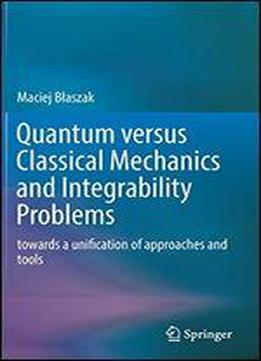# Quantum Versus Classical Mechanics And Integrability Problems: Towards A Unification Of Approaches And Tools by Maciej BЕ‚aszak / 2019 / English / PDF

This accessible monograph introduces physicists to the general relation between classical and quantum mechanics based on the mathematical idea of deformation quantization and describes an original approach to the theory of quantum integrable systems developed by the author.The first goal of the book is to develop of a common, coordinate free formulation of classical and quantum Hamiltonian mechanics, framed in common mathematical language.In particular, a coordinate free model of quantum Hamiltonian systems in Riemannian spaces is formulated, based on the mathematical idea of deformation quantization, as a complete physical theory with an appropriate mathematical accuracy.The second goal is to develop of a theory which allows for a deeper understanding of classical and quantum integrability. For this reason the modern separability theory on both classical and quantum level is presented. In particular, the book presents a modern geometric separability theory, based on bi-Poissonian and bi-presymplectic representations of finite dimensional Liouville integrable systems and their admissible separable quantizations.The book contains also a generalized theory of classical Stckel transforms and the discussion of the concept of quantum trajectories.In order to make the text consistent and self-contained, the book starts with a compact overview of mathematical tools necessary for understanding the remaining part of the book. However, because the book is dedicated mainly to physicists, despite its mathematical nature, it refrains from highlighting definitions, theorems or lemmas.Nevertheless, all statements presented are either proved or the reader is referred to the literature where the proof is available.

views: 390